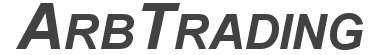Home | Free Email Newsletter | Contact
 Beginnings   New Traders   Compounding   Starting Capital   Stock Entry   Expectancy Money Management    Money Management   Kelly Formula Newsletter    Free Weekly Newsletter   Daily Newsletter E-Books   Three Day Hammer   Trader's Bible   Trading Systems System Development   Where to Start   Trading Systems Hall of Fame    Richard Wyckoff   Jesse Livermore Resources    Reading List   Unclaimed Money Company Info    Contact  Free Newsletter Receive our weekly list of  3 Day Hammer Stocks   First Name   Email
 The Kelly Formula The exact amount to risk on a trade is the big question in all money management systems. Risk too little will have your money won’t grow. Risk too much and the drawdown will put you out of business. In between too little and too much risk is an area where capital growth will grow to its maximum potential. The original Kelly Formula was developed back in 1956 to solve a problem involving random interference on telephone lines. What Kelly discovered was a method of increasing data flow while reducing random information loss. Before calculating the optimum percent to risk, you need your winning percentage (W%), the average size of your winning trades(W) and the average size of your losing trades(L). The basic Kelly formula can be calculated as: Optimum Risk Percent = W% – [(1-W%)/(W/L)] Let’s have an example. Suppose you have a system that has a winning percentage of 0.6. Your system also has average winning trade of 8 and your average loss is 4. Thus, W% = 0.6 and W = 8 and L = 4. Using these numbers results in the following: Optimum Risk Percent = 0.6 – [(1 – 0.6)/(8/4] = 0.6 – [0.4/2] =0.6 – 0.2 =0.4 Thus, the percentage of equity that would provide a maximum rate of return is 40%. The major problem with the Kelly formula is drawdown. If you have a system that is right 60% of the time, you could still be wrong 10 or even 15 times in a row sometime during your lifetime of trading. Risking too high a percentage would be disastrous. The Kelly formula implies (sorry, you have to read the original report) that unless your drawdown is less than 25%, never risk more than 25% of you equity. The Kelly Formula is critical for traders wanting optimal rates of return. For practical application of the Kelly Formula, use 80% of the Kelly %. In the above example – we derived an optimum risk size of 40% of capital. This is too high for practical use. Instead, we would use 80% of 25% which is equal to 20%. Determine how many trades you are likely to have on at one time and then divide your 80%-Kelly value by that number of trades. For example, if you are likely to have as many as 8 trades at one time, then your optimal trading size, using the above example, would be [20%/8] or about 2.5% of your trading equity.The math behind the system is pretty complicated. You may also want to read more about Kelly Criterion in two books written by Dr. Edward O. Thorp THE MATHEMATICS OF GAMBLING THE KELLY CRITERION IN BLACKJACK, SPORTS BETTING, AND THE STOCK MARKET